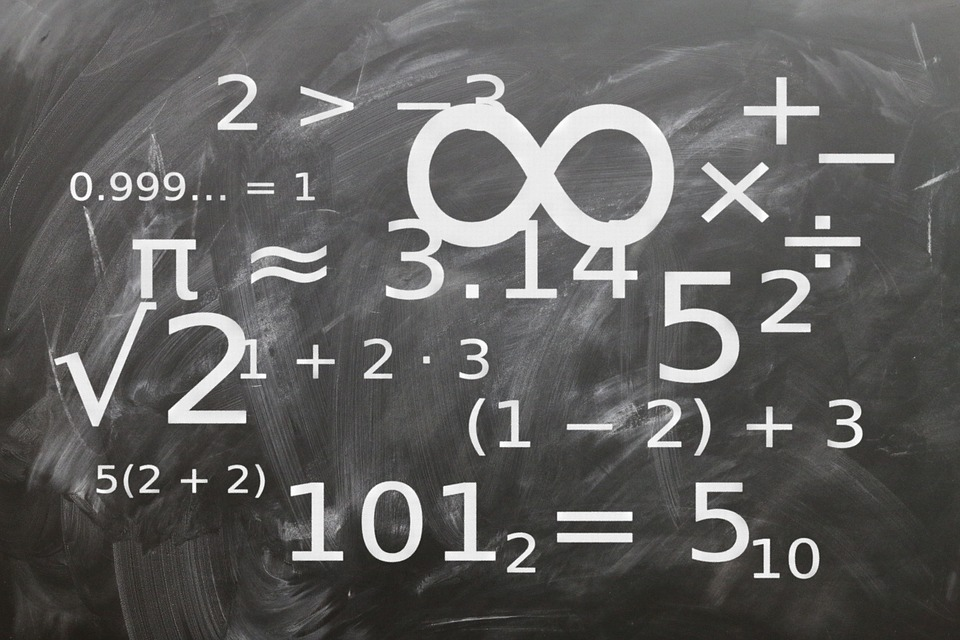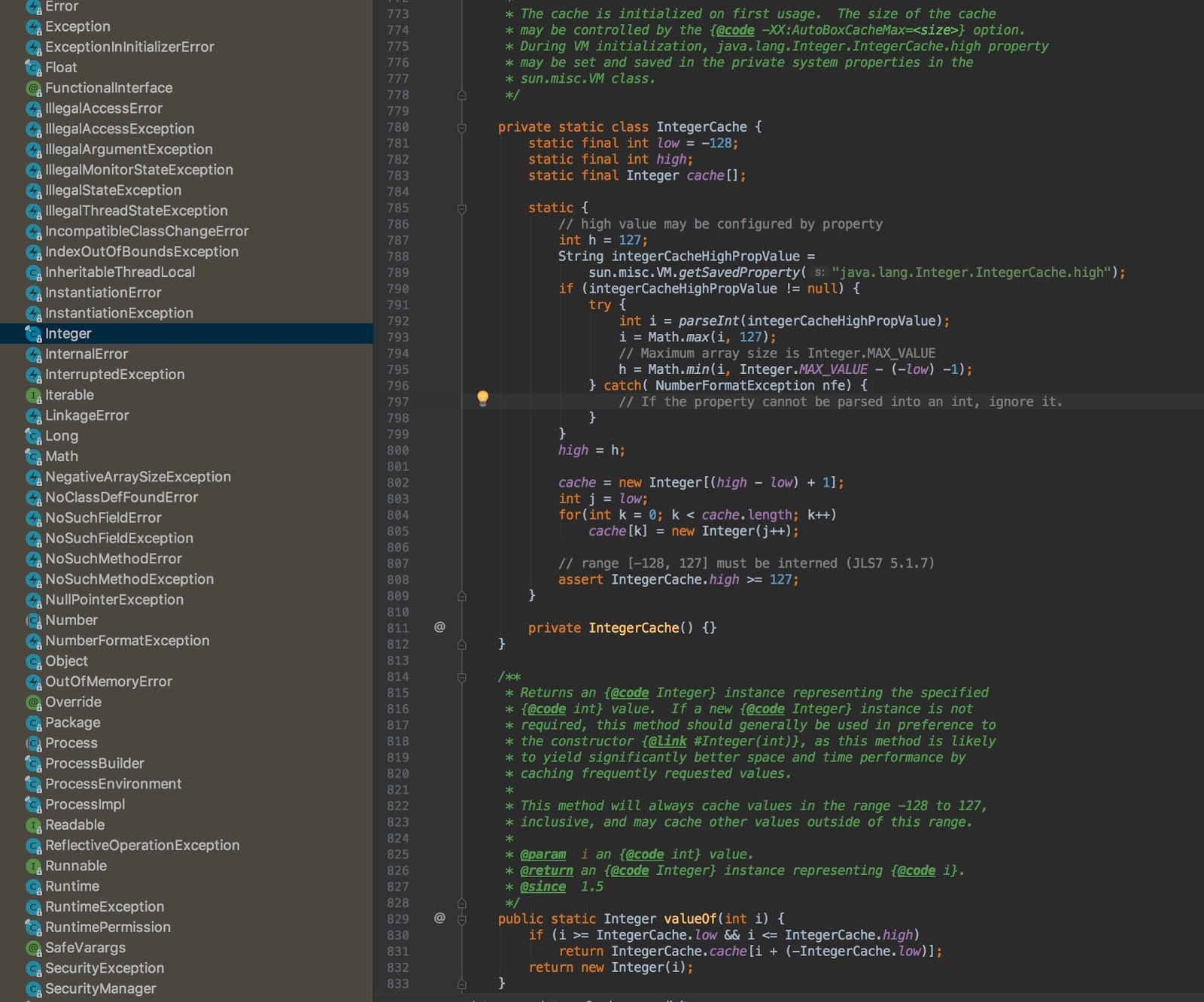{{announcement.body}}
{{announcement.title}}

# Java Integer Cache: Why Integer.valueOf(127) == Integer.valueOf(127) Is True

DZone 's Guide to

# Java Integer Cache: Why Integer.valueOf(127) == Integer.valueOf(127) Is True

### So here's the cache...

· Java Zone ·
Free Resource

Comment (0)

Save
{{ articles.views | formatCount}} ViewsIn an interview, one of my friends was asked: If we have two Integer objects, `Integer a = 127; Integer b = 127;` Why does `a == b` evaluate to `true` when both are holding two separate objects? In this article, I will try to answer this question and explain the answer.

You may also like: The Internal Cache of Integers

The short answer to this question is, direct assignment of an `int` literal to an `Integer` reference is an example of auto-boxing concept where the literal value to object conversion code is handled by the compiler, so during compilation phase compiler converts `Integer a = 127;` to `Integer a = Integer.valueOf(127);`.

The `Integer` class maintains an internal `IntegerCache` for integers which, by default, ranges from `-128 to 127` and `Integer.valueOf()` method returns objects of mentioned range from that cache. So `a == b` returns true because `a` and `b` both are pointing to the same object.

In order to understand the short answer, let's first understand the Java types, all types in Java lies under two categories

1. Primitive Types: There are 8 primitive types (`byte``short``int``long``float``double``char`, and `boolean`) in Java, which holds their values directly in the form of binary bits.
For example, `int a = 5; int b = 5;` here `a` and `b` directly holds the binary value of 5, and if we try to compare `a` and `b` using `a == b`, we are actually comparing `5 == 5`, which returns true.
2. Reference Types: All types other than primitive types lies under the category of reference types, e.g. Classes, Interfaces, Enums, Arrays, etc. and reference types holds the address of the object instead of the object itself.
For example, `Integer a = new Integer(5); Integer b = new Integer(5)`, here, a and b do not hold the binary value of `5` instead `a` and `b` holds memory addresses of two separate objects where both objects contain a value `5`. So if we try to compare `a` and `b` using `a == b, ,`we are actually comparing those two separate memory addresses. Hence, we get `false`, to perform actual equality on `a` and `b` we need to perform `a.euqals(b)`.
Reference types are further divided into 4 categories:  Strong, Soft, Weak and Phantom References.
And we know that Java provides wrapper classes for all primitive types and support auto-boxing and auto-unboxing.
``````// Example of auto-boxing, here c is a reference type
Integer c = 128; // Compiler converts this line to Integer c = Integer.valueOf(128);

// Example of auto-unboxing, here e is a primitive type
int e = c; // Compiler converts this line to int e = c.intValue();``````

Now, if we create two integer objects `a` and `b,` and try to compare them using the equality operator `==`, we will get `false` because both references are holding different-different objects

``````Integer a = 128; // Compiler converts this line to Integer a = Integer.valueOf(128);
Integer b = 128; // Compiler converts this line to Integer b = Integer.valueOf(128);

System.out.println(a == b); // Output -- false``````

But if we assign the value `127` to both `a` and `b` and try to compare them using the equality operator `==`, we will get `true` why?

``````Integer a = 127; // Compiler converts this line to Integer a = Integer.valueOf(127);
Integer b = 127; // Compiler converts this line to Integer b = Integer.valueOf(127);

System.out.println(a == b); // Output -- true
``````

As we can see in the code, we are assigning different objects to `a` and `b` but `a == b` can return true only if both `a` and `b` are pointing to the same object.

So, how does the comparison return true? what's actually happening here? are `a` and `b` pointing to the same object?

Well, until now, we know that the code `Integer a = 127;` is an example of auto-boxing and compiler automatically converts this line to `Integer a = Integer.valueOf(127);`.

So, it is the `Integer.valueOf()` method that is returning these integer objects, which means this method must be doing something under the hood.

And if we take a look at the source code of `Integer.valueOf()` method, we can clearly see that if the passed int literal `i` is greater than `IntegerCache.low` and less than `IntegerCache.high ,`then the method returns Integer objects from `IntegerCache`. Default values for `IntegerCache.low` and `IntegerCache.high` are `-128` and `127` respectively.

In other words, instead of creating and returning new integer objects, `Integer.valueOf()` method returns Integer objects from an internal `IntegerCache` if the passed `int` literal is greater than `-128` and less than `127`.

``````/**
* Returns an {@code Integer} instance representing the specified
* {@code int} value.  If a new {@code Integer} instance is not
* required, this method should generally be used in preference to
* the constructor {@link #Integer(int)}, as this method is likely
* to yield significantly better space and time performance by
* caching frequently requested values.
*
* This method will always cache values in the range -128 to 127,
* inclusive, and may cache other values outside of this range.
*
* @param  i an {@code int} value.
* @return an {@code Integer} instance representing {@code i}.
* @since  1.5
*/
public static Integer valueOf(int i) {
if (i >= IntegerCache.low && i <= IntegerCache.high)
return IntegerCache.cache[i + (-IntegerCache.low)];
return new Integer(i);
}``````

Java caches integer objects that fall into -128 to 127 range because this range of integers gets used a lot in day-to-day programming, which indirectly saves some memory.

As you can see in the following image, the `Integer` class maintains an inner static `IntegerCache` class, which acts as the cache and holds integer objects from -128 to 127, and that's why when we try to get integer object for `127,` we always get the same object.The cache is initialized on the first usage when the class gets loaded into memory because of the `static block`. The max range of the cache can be controlled by the `-XX:AutoBoxCacheMax` JVM option.

This caching behavior is not applicable to `Integer` objects only. Similar to `Integer.IntegerCache`, we also have `ByteCache`, `ShortCache`, `LongCache`, `CharacterCache` for `Byte`, `Short`, `Long`, `Character` respectively.

`Byte``Short`, and `Long` have a fixed range for caching between -127 to 127 (inclusive), but for `Character`, the range is from 0 to 127 (inclusive). The range can be modified via argument only for Integer but not for others.

The Internal Cache of Integers

Java Concurrency: AtomicInteger

Topics:
btye, cache, integer cache, java, java integer, long, short

Comment (0)

Save
{{ articles.views | formatCount}} Views

Published at DZone with permission of Naresh Joshi , DZone MVB. See the original article here.

Opinions expressed by DZone contributors are their own.